• 设置横坐标字体大小代码： plt.xticks(fontsize=15) 设置纵坐标字体大小代码： plt.yticks(fontsize=15)
设置横坐标字体大小代码：
plt.xticks(fontsize=15)

设置纵坐标字体大小代码：
plt.yticks(fontsize=15)



展开全文横坐标
• 设置输出的图片大小： figsize = 11,9 figure, ax = plt.subplots(figsize=figsize) 画简单的折线图，同时标注线的形状、名称、粗细： A,=plt.plot(x1,y1,'-r',label='A',linewidth=5.0,ms=10) ...
设置输出的图片大小：

figsize = 11,9
figure, ax = plt.subplots(figsize=figsize)

画简单的折线图，同时标注线的形状、名称、粗细：

A,=plt.plot(x1,y1,'-r',label='A',linewidth=5.0,ms=10)

其中线条样式以及颜色设置可参考：https://blog.csdn.net/code_segment/article/details/79217700，个人觉得介绍非常详尽。

线条粗细使用linewidth设置，对应线条上的marker大小设置为ms参数。因为有时候粗线条，所以对应marker大小也需要增加。

如果想要标记marker为空心，可以在后面加上 markerfacecolor='none'

设置图例以及对应属性：

legend = plt.legend(handles=[A,B],prop=font1)

图例的字体格式在prop中进行设置，赋值font1可以是一个字典，包含各个属性及其对应值，属性包括family（字体）、size（字体大小）等常用属性，更详细的解释可参考matplotlib手册中关于legend prop的解释。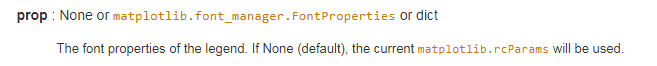一种比较简单的设置为：

font1 = {'family' : 'Times New Roman',
'weight' : 'normal',
'size'   : 23,
}

坐标轴刻度密度/间隔设置：

ax.xaxis.set_major_locator(MultipleLocator(10))

括号中的数字为对应的刻度间隔值，y轴对应类似。

坐标轴刻度值属性设置：

plt.tick_params(labelsize=23)
labels = ax.get_xticklabels() + ax.get_yticklabels()
[label.set_fontname('Times New Roman') for label in labels]

其中tick_params中可设置一系列属性，包括刻度值字体大小、方向、大小，颜色等一系列属性，具体可参见手册中关于tick_params的解释。

比较特殊的是，其中并没有对刻度值的字体进行设置的属性，所以我们需要使用下面两行进行设置，在最初使用plt.subplots中有得到一个返回值ax，我们使用ax.get_xticklabels()以及ax.get_yticklabels()来得到所有的刻度值，并使用set_fontname函数来设置属性。

坐标轴名称以及对应字体属性设置：

plt.xlabel('round',font2)
plt.ylabel('value',font2)

这种比较简单，第一个参数为坐标轴名称，第二个参数也是一个字典参数，和上文提及的dict font1格式相同。

有时候，因为调整了坐标刻度的字体大小，影响了坐标轴label的显示。所以我们需要通过调整坐标轴边距来显示label

plt.subplots_adjust(left = 0.15,bottom=0.128)

下面我们给出一个比较简单的画图过程:

#--coding:utf-8--
import  matplotlib.pyplot as plt

#数据设置
x1 =[0,5000,10000, 15000, 20000, 25000, 30000, 35000, 40000, 45000, 50000, 55000];
y1=[0, 223, 488, 673, 870, 1027, 1193, 1407, 1609, 1791, 2113, 2388];

x2 =[0,5000,10000, 15000, 20000, 25000, 30000, 35000, 40000, 45000, 50000, 55000];
y2=[0, 214, 445, 627, 800, 956, 1090, 1281, 1489, 1625, 1896, 2151];

#设置输出的图片大小
figsize = 11,9
figure, ax = plt.subplots(figsize=figsize)

#在同一幅图片上画两条折线
A,=plt.plot(x1,y1,'-r',label='A',linewidth=5.0)
B,=plt.plot(x2,y2,'b-.',label='B',linewidth=5.0)

#设置图例并且设置图例的字体及大小
font1 = {'family' : 'Times New Roman',
'weight' : 'normal',
'size'   : 23,
}
legend = plt.legend(handles=[A,B],prop=font1)

#设置坐标刻度值的大小以及刻度值的字体
plt.tick_params(labelsize=23)
labels = ax.get_xticklabels() + ax.get_yticklabels()
[label.set_fontname('Times New Roman') for label in labels]

#设置横纵坐标的名称以及对应字体格式
font2 = {'family' : 'Times New Roman',
'weight' : 'normal',
'size'   : 30,
}
plt.xlabel('round',font2)
plt.ylabel('value',font2)

#将文件保存至文件中并且画出图
plt.savefig('figure.eps')
plt.show()

最终生成的图片效果如下：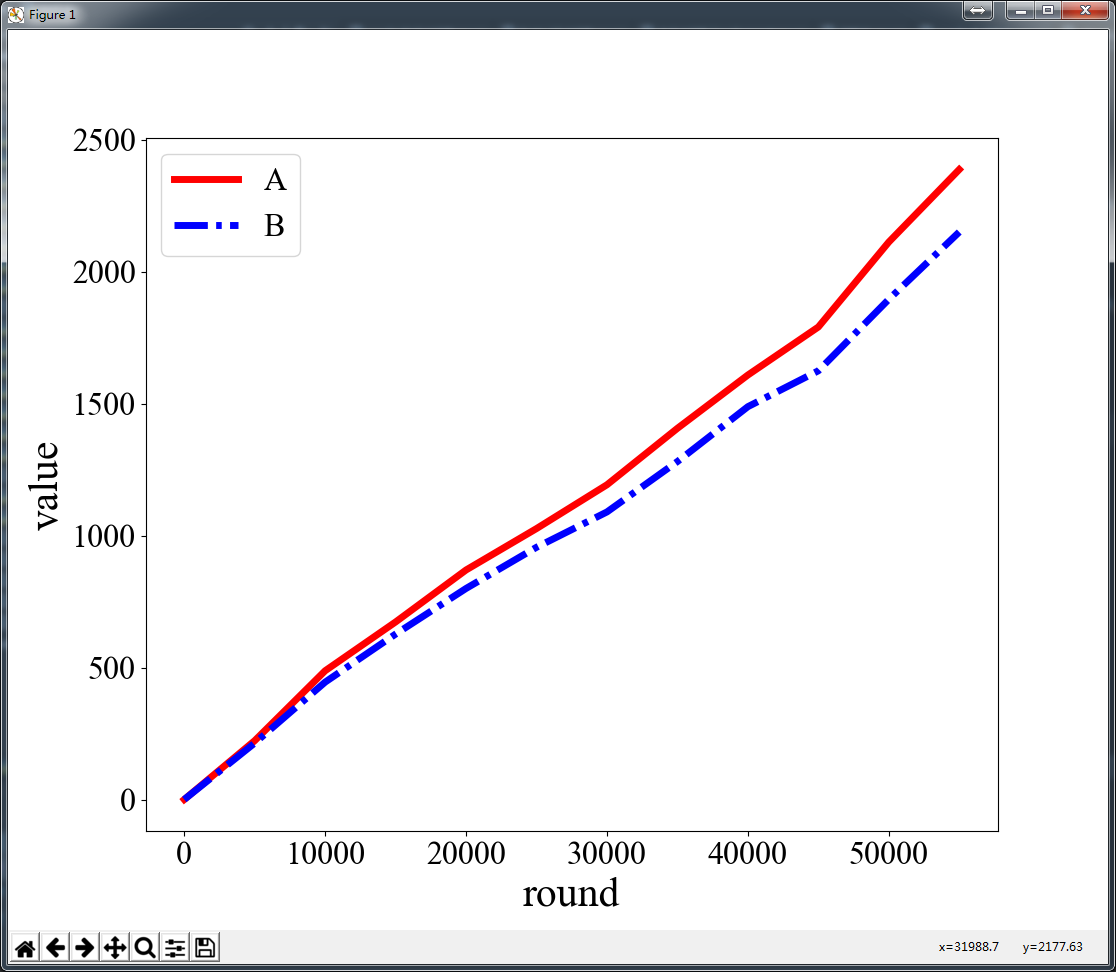更多的画图代码参考也可见手册中的Examples using matplotlib.pyplot.plot，可以翻到超链接跳转页面的最下方，有很多画图的示例，点进去即为对应实现代码。可以找到适用的代码实现方式。pyplot.subplots下也有很多很好的示例！

展开全文matplotlib
• python很火，因为有各种库的支持，所以功能格外强大。在可视化方面，目前用得较多的是matplotlib. 在基于matplotlib.pyplot画带色标(colorbar)的图时候，往往为了美观和科研用途，需要对colorbar的Ticks(刻度) ，...

介绍
python很火，因为有各种库的支持，所以功能格外强大。在可视化方面，目前用得较多的是matplotlib.
在基于matplotlib.pyplot画带色标(colorbar)的图时候，往往为了美观和科研用途，需要对colorbar的Ticks(刻度) ，标签(label)和fonddict(字体进行设置)。但是很多初学者都苦于这些东西的设置，因为太麻烦了(别问我怎么知道的)。以下将介绍有用的方法来解决这些问题。

import matplotlib.pyplot as plt


解决问题

下面将分两种情况来进行介绍
情况1：当colorbar在需要额外指定时(即不能自动生成)。
这种情况最为常见。如 plt.contourf  ,plt.imshow, 等。即就是先基于plt.contourf生成图后，再获取该图的句柄，再设置colorbar。画主图和colorbar是两个语句。
还是举个例子吧

List item

import numpy as np
import matplotlib.pyplot as plt
#设置一个随机种子,
#生成固定数值的随机整数数组
seed=np.random.seed(42)
data=np.random.randint(0,10,size=(10,10))

fig=plt.figure(figsize=(10,8))
#这就是所谓的第一种情况哦
h=plt.contourf(data)
cb=plt.colorbar(h)

生成的图如下所示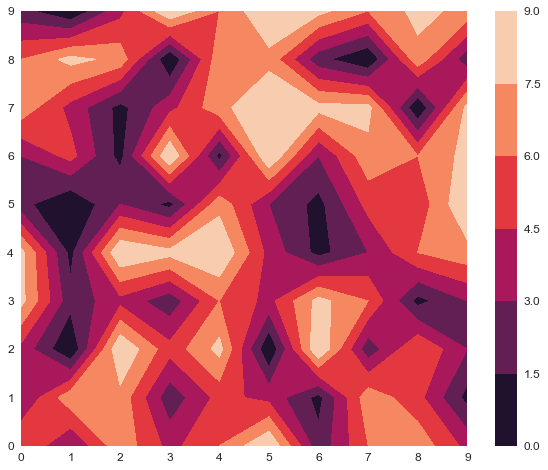List item

我们设置一下主图的横纵坐标的刻度字体大小
plt.xticks(fontsize=16)
plt.yticks(fontsize=16)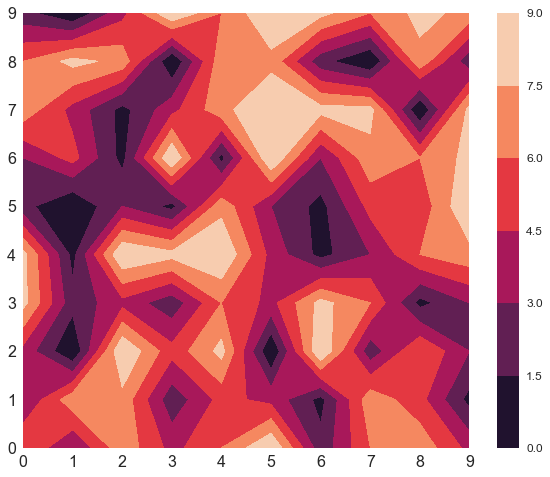看，在这种情况下，色标的刻度大小与主图的刻度大小不符合，这是完全不符合一个合格的程序员的审美的。因此需要更改colorbar刻度字体大小。

List item

fig=plt.figure(figsize=(10,8))
#这就是所谓的第一种情况哦
h=plt.contourf(data)
cb=plt.colorbar(h)
cb.ax.tick_params(labelsize=16)  #设置色标刻度字体大小。
plt.xticks(fontsize=16)
plt.yticks(fontsize=16)
font = {'family' : 'serif',
'color'  : 'darkred',
'weight' : 'normal',
'size'   : 16,
}
cb.set_label('colorbar',fontdict=font) #设置colorbar的标签字体及其大小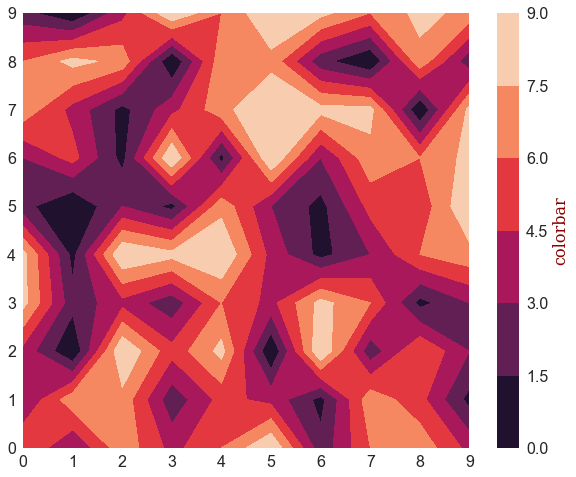这个图是不是漂亮多啦

List item

当你想改变colorbar刻度数值大小时，可以采用cb.set_ticks, cb.set_ticklabels
当然你也可以获取其刻度大小，可以用cb.get_ticks。还有cb.get_cmap可以获取颜色属性。

List item
当你需要进行其他操作，如设置颜色，大小限制什么的，可以自己随意上手。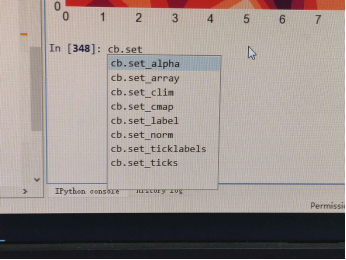综上，最重要的一句是  cb.ax.tick_params(labelsize=16)。其他的属性也可以 用 cb.ax.+table键进行查看。
情况2：当colorbar可以在生成主图时就自动生成时
这种情况在生成热图时较为常见。
可以用seaborn来生成热图(heatmap)
import seaborn as sns
import matplotlib.pyplot as plt
import numpy as np
seed=np.random.seed(42)
data=np.random.randint(0,10,size=(10,10))

fig=plt.figure(figsize=(10,8))
h=sns.heatmap(data, annot=True,fmt='d',linewidths=0.5)

生成图如下：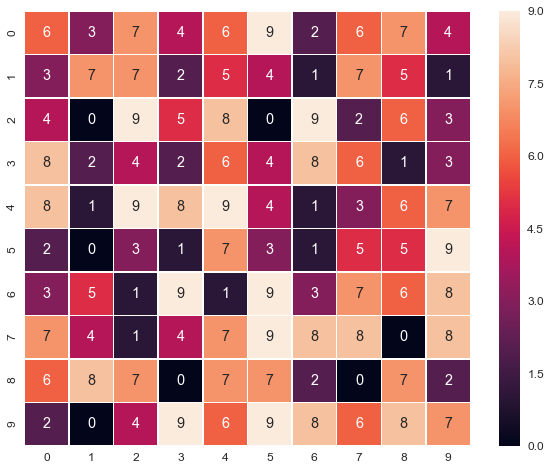heatmap的各个参数介绍可见如下链接：
http://seaborn.pydata.org/generated/seaborn.heatmap.html
https://blog.csdn.net/sunchengquan/article/details/78573244
https://blog.csdn.net/m0_38103546/article/details/79935671
heatmap参数中，默认cbar=True,即默认画出colorbar，其中cbarkws为一个字典， 可以用来设置colorbar的一些属性，包括 thrink, orentation等 。但是我没有找到cbrkws可以设置哪些属性以及怎么设置。
因此这里不利用cbrkws进行colorbar设置。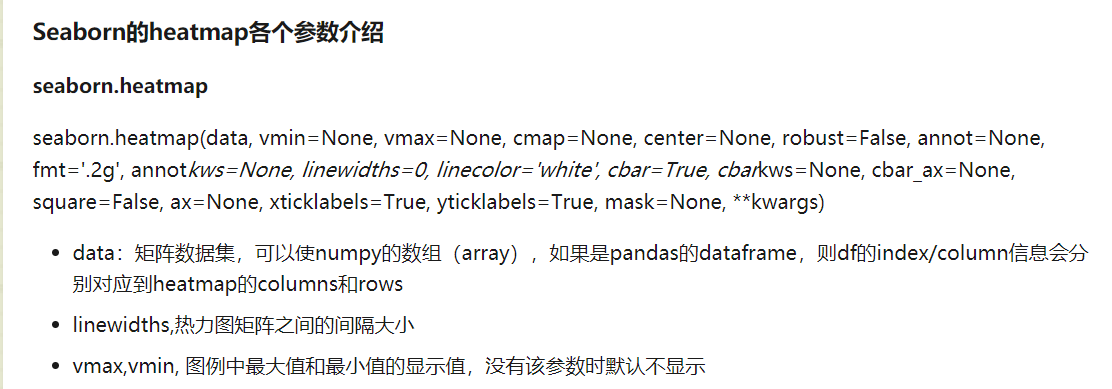fig=plt.figure(figsize=(10,8))
h=sns.heatmap(data, annot=True,fmt='d',linewidths=0.5,cbar=False) #设置不使用其默认自带的colorbar
cb=h.figure.colorbar(h.collections) #显示colorbar
cb.ax.tick_params(labelsize=16) #设置colorbar刻度字体大小。
plt.xticks(fontsize=16)
plt.yticks(fontsize=16)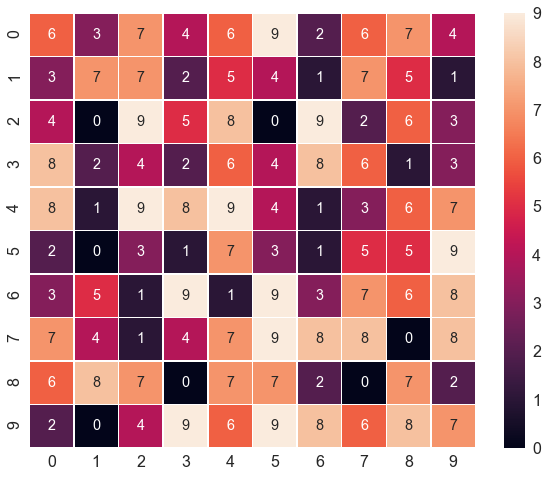其他如标签和刻度范围和色标的操作同第一种情况。
参考链接：
https://blog.csdn.net/henkekao/article/details/72864564
https://stackoverflow.com/questions/37233108/seaborn-change-font-size-of-the-colorbar
https://stackoverflow.com/questions/34820239/seaborn-heatmap-colorbar-label-as-percentage


展开全文• 本篇文章主要介绍了python Matplotlib画图之调整字体大小的示例，小编觉得挺不错的，现在分享给大家，也给大家做个参考。一起跟随小编过来看看吧
• x,y 轴刻度 plt.xticks(xticks,xtickslabel,fontsize = 15,alpha=2.0)#,rotation=60) plt.yticks(fontsize=10) 2 x,y ；label plt.ylabel('Height (km)'+str(ye),fontsize=10) ... ...


x,y 轴刻度

     plt.xticks(xticks,xtickslabel,fontsize = 15,alpha=2.0)#,rotation=60)
plt.yticks(fontsize=10)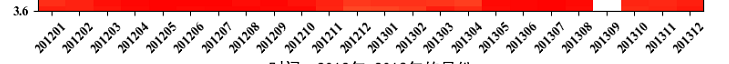2  x,y ；label

		plt.ylabel('Height  (km)'+str(ye),fontsize=10)


plt.colorbar 刻度

        cbr=plt.colorbar()#orientation='horizontal')
cbr.ax.tick_params(labelsize=20)


4 plt .colorbar     label

    font = {'family': 'serif',
'color': 'darkred',
'weight': 'normal',
'size': 18,
}
cbr.set_label(unit,fontdict=font)展开全文• import matplotlib.pyplot as plt plt.xticks([0, 100, 200, 300, 400, 500, 600, 700]) plt.tick_params(labelsize=13) #刻度字体大小13
• 在我们的画图函数里直接设置即可 1.3 字体大小的设置 如果我们想设置我们所画的图片，全局字体的大小，就是设置统一的大小，我们可以这么做： 全局字体的大小设置 matplotlib.rcParams.update({'font.size': 12}) ...
• import matplotlib.pyplot as plt ...plt.title('Input',fontdict={'weight':'normal','size': 20}) #改变图标题字体 plt.xlabel('Time', fontdict={'weight': 'normal', 'size': 13})#改变坐标轴标题字体 ...
• python绘图的包大家应该不会陌生，但是，对图的常规设置不一定会知道（其实自己也是才知道的），比如：坐标轴的字体大小、颜色设置；标题的字体颜色大小设置；线的粗细、颜色；图片风格的设置等。了解这些常规设置...
• python绘图的包大家应该不会陌生，但是，对图的常规设置不一定会知道（其实自己也是才知道的），比如：坐标轴的字体大小、颜色设置；标题的字体颜色大小设置；线的粗细、颜色；图片风格的设置等。了解这些常规设置...# python画图字体大小python 订阅